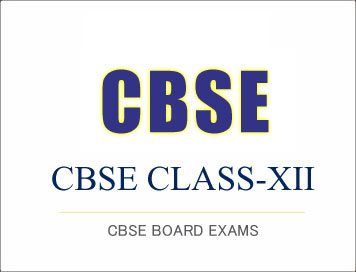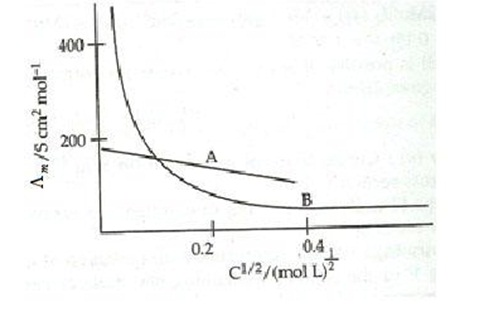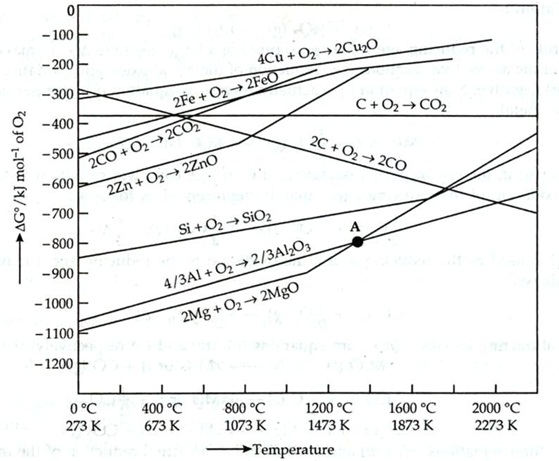# (Download) CBSE Class-12 Sample Paper And Marking Scheme 2015-16 : Chemistry

Disclaimer: This website is not at associated with CBSE, For official website of CBSE visit - www.cbse.nic.in

### (Download) CBSE Class-12 Sample Paper And Marking Scheme 2015-16 : Chemistry

CHEMISTRY
SAMPLE QUESTION PAPER

CLASS XII

Time: 3 hrs    MM: 70

GENERAL INSTRUCTIONS:
1.    All the questions are compulsory.
2.    Q. No. 1 to 5 are very short answer type, carrying 1mark each.
3.    Q. No. 6 to 10 are short answer type, carrying 2 marks each.
4.    Q. No. 11 to 22 are short answer type, carrying 3 marks each.
5.    Q.No.23 is a value based question carrying 4 marks.
6.    Q. No. 24 to 26 are long answer type, carrying 5 marks each.
7.    Use of calculators is not allowed, use log tables wherever required.

1.  Name the non stoichiometric point defect responsible for colour in alkali metal halides.

2.  What is shape selective catalysis?

3.  Amongst the isomeric alkanes of molecular formula C5H12, identify the one that on photochemical chlorination yields a single monochloride.

4.  Give the IUPAC name and structure of the amine obtained when 3-chlorobutanamide undergoes Hoffmann –bromamide reaction.

5.    How many ions are produced from the complex,[Co (NH3)6]Cl2 in solution?

6.  Will the elevation in boiling point be same if 0.1 mol of Sodium chloride or 0.1 mol of sugar is dissolved in 1L of water? Explain.

7.  The following curve is obtained when molar conductivity ( L m ) is plotted against the square    root    of    concentration,    c1/2    for    two    electrolytes    A    and    B(a) How do you account for the increase in the molar conductivity of the electrolyte A on dilution.

(b) As  seen  from  the  graph,  the  value  of  limiting  molar  conductivity  ( o m ) for electrolyte B cannot be obtained graphically. How can this value be obtained?

8.  Name the following:
(a) A  transition  metal  which  does  not  exhibit  variation  in  oxidation  state  in  its compounds.
(b) A compound where the transition metal is in the +7 oxidation state.
(c) A member of the lanthanoid series which is well known to exhibit +4 oxidation state.
(d) Ore used in the preparation of Potassium dichromate.

9.  Arrange the following in order of property indicated for each set: (a) F2, Cl2, Br2, I2  – increasing bond dissociation enthalpy
(b) PH3, AsH3, BiH3, SbH3, NH3  – increasing base strength

10. (a)    Predict the major product of acid catalysed dehydration of 1-Methylcyclohexanol.

(b)  You are given benzene, conc.H2SO4, NaOH and dil.HCl. Write the preparation of phenol using these reagents.

OR
Draw the structures of any two isomeric alcohols (other than 1o  alcohols) having molecular formula C5H12O and give their IUPAC names.

11. An element occurs in the bcc structure with cell edge of 288 pm. The density of the element is 7.2  g  cm-3. How many atoms of the element does 208g of the element contain?

12. Calculate the boiling point of a 1M aqueous solution (density 1.04 g mL-1) of Potassium chloride (Kb for water = 0.52 K kg mol-1, Atomic masses: K=39u, Cl=39.9u)
Assume, Potassium chloride is completely dissociated in solution

13. A galvanic cell consists of a metallic zinc plate immersed in 0.1M Zn(NO3)2  solution and metallic plate of lead in 0.02M Pb(NO3)2  solution. Calculate the emf of the cell. Write the chemical equation for the electrode reactions and represent the cell.

(Given:Eo Zn 2+ / Zn = -0.76V ;  Eo Pb2+ / Pb  = -0.13V )

(a) What happens when a freshly precipitated Fe (OH)3  is shaken with a little amount of dilute solution of FeCl3?
(b) Why are lyophilic colloidal sols more stable than lyophobic colloidal sols?

(c) What form Freundlich adsorption equation will take at high pressure?

15. What chemical principle is involved in choosing a reducing agent for getting the metal from its oxide ore?

Consider the metal oxides, Al2O3  and FeO and justify the choice of reducing agent in each case.OR

Account for the following facts:
(a) the reduction of a metal oxide is easier if the metal formed is in the liquid state at the temperature of reduction.
(b) Limestone is used in the manufacture of pig iron from haematite.
(c) Pine oil is used in the froth floatation process used to concentrate sulphide ores.

16. (i)    For M2+/M and M3+/M2+ systems, Eo values for some metals are as follows:

Cr2+/Cr = −0.9V    Cr3+/Cr2+ = −0.4V
Mn2+/Mn = −1.2V    Mn3+/Mn2+ = +1.5V

Fe2+/Fe   = −0.4V    Fe3+/Fe2+ = +0.8V

Use this data to comment upon
(a) the stability of Fe3+ in acid solution as compared to that of Cr3+ and Mn3+
(b) the ease with which iron can be oxidised as compared to the similar process for either Cr or Mn metals
(ii)    What can be inferred from the magnetic moment of the complex K4[Mn(CN)6] Magnetic moment: 2.2 BM?

17. (i)    Describe the type of hybridisation for the complex ion [Fe(H2O)6]2+.
(ii)    Write the IUPAC name of the ionisation isomer of the coordination compound
[Co(NH3)5Br]SO4.  Give  one  chemical  test  to  distinguish  between  the  two compounds.